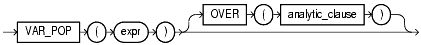## VAR_POP

#### Syntax

var_pop::=Text description of var_pop

#### Purpose

`VAR_POP` returns the population variance of a set of numbers after discarding the nulls in this set. You can use it as both an aggregate and analytic function.

The `expr` is a number expression, and the function returns a value of type `NUMBER`. If the function is applied to an empty set, then it returns null. The function makes the following calculation:

```(SUM(expr2) - SUM(expr)2 / COUNT(expr)) / COUNT(expr)

```
 See Also: "Aggregate Functions" "About SQL Expressions" for information on valid forms of `expr`

#### Aggregate Example

The following example returns the population variance of the salaries in the `employees` table:

```SELECT VAR_POP(salary) FROM employees;

VAR_POP(SALARY)
---------------
15140307.5
```

#### Analytic Example

The following example calculates the cumulative population and sample variances of the monthly sales in 1998:

```SELECT t.calendar_month_desc,
VAR_POP(SUM(s.amount_sold))
OVER (ORDER BY t.calendar_month_desc) "Var_Pop",
VAR_SAMP(SUM(s.amount_sold))
OVER (ORDER BY t.calendar_month_desc) "Var_Samp"
FROM sales s, times t
WHERE s.time_id = t.time_id AND t.calendar_year = 1998
GROUP BY t.calendar_month_desc;

CALENDAR    Var_Pop   Var_Samp
-------- ---------- ----------
1998-01           0
1998-02  6.1321E+11 1.2264E+12
1998-03  4.7058E+11 7.0587E+11
1998-04  4.6929E+11 6.2572E+11
1998-05  1.5524E+12 1.9405E+12
1998-06  2.3711E+12 2.8453E+12
1998-07  3.7464E+12 4.3708E+12
1998-08  3.7852E+12 4.3260E+12
1998-09  3.5753E+12 4.0222E+12
1998-10  3.4343E+12 3.8159E+12
1998-11  3.4245E+12 3.7669E+12
1998-12  4.8937E+12 5.3386E+12
```